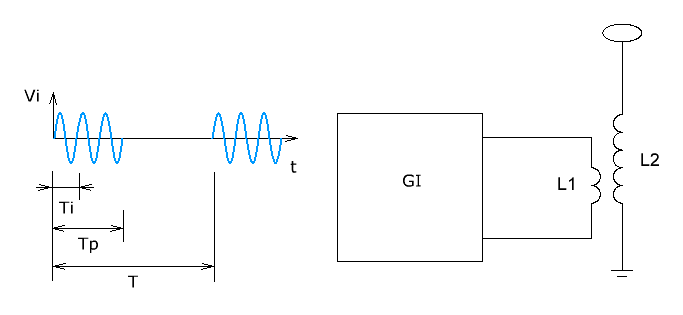Research website of Vyacheslav Gorchilin
Calculation of pulse pumping Tesla transformer
Duty cycle: 1 .. 2000
Frequency ratio: 1 .. 24
Time constant L/R: 0.001 .. 1
The result
The amplitude of the first harmonic: 2.45*10-3
The effective value of the amplitude of the first harmonic: 1.73*10-3
The effective value of the amplitude of the driving frequency: 5*10-1
The ratio of the effective values: 0.0035
The oscillation frequency of the oscillator, kHz => duration of packs of pulses (pulse a), the NSEC
Share
Detail
In order to share in your project, you need to copy the link and paste it into a blog, forum or another website:
In this calculator you can calculate some of the data schema of a classical pumping Tesla transformer (TT). It is assumed that TT is excited with a generator of the pulse train GI (see figure). Specifies the (primary) frequency is the value inverse to Ti, the modulation frequency — the reciprocal to T, and duty cycle — as T/Tp. An important parameter is the ratio of two frequencies: the modulating and driving, which is T/Ti. The time constant TT of the secondary winding L2 is determined by parameters of inductance and active resistance, and is as L/R.
The left graph shows the response of the secondary winding L2 is excited, the right graph — its spectrum. All values for ease of display, relative. For example, the period of the modulating oscillations T and amplitude Vi is equal to one.The calculation is relative to the first harmonic from the spectrum obtained in the L2 hesitation. This will remove the useful power to the load without affecting the wave processes in the TT. The result is the amplitude of the first harmonic and effective (RMS) amplitudes of the harmonics of the driving frequency. The main result is the ratio of last two quantities. With proper selection of initial parameters, this result can be greater than unity, and a power ratio which is as the square of this magnitude — much greater than unity!
In the "advanced settings" you can enter a value that specifies the frequency of the generator and get the value for the time duration of the pulse packet. This is especially useful for counting bundles with one pulse. Example.
The most complete explanation of this calculation see here. For fans of MathCAD and you can download a complete analog of this calculator.